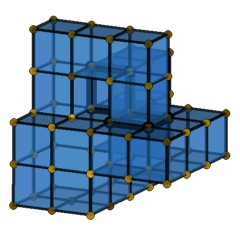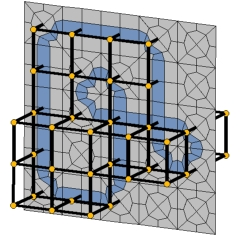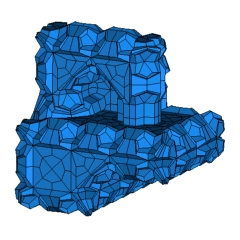A Cubical 4-Polytope with an Odd Number of FacetsElectronic Geometry Model No. 2004.07.001

Authors

Alexander Schwartz and Günter M. Ziegler

Description

A cubical 4-polytope with an odd number of facets and a dual Boy's surface.

It has been observed by Stanley and by MacPherson that every cubical d-polytope determines a PL immersion of an abstract cubical (d-2)-manifold into (the barycentric subdivision of) the boundary of the polytope . In the case of cubical 4-polytopes each connected component of the dual manifold is a surface (a compact 2-manifold without boundary).

We prove in  that every normal crossing codimension one immersion of a compact 2-manifold into R3 is PL-equivalent to a dual manifold immersion of a cubical 4-polytope. Thus, in particular non-orientable dual 2-manifolds do arise: In  we described a rather small and simple instance of a 4-polytope with a non-orientable dual 2-manifold (one component is a Klein bottle). If the dual two-manifold has odd Euler characteristic (with an odd number of non-orientable components of odd genus), the resulting cubical 4-polytope has an odd number of facets.

Here we present an example of a cubical 4-polytope with an odd number of facets, which has Boy surface (an immersed projective plane with a single triple point) as a dual manifold immersion. This solves problems of Eppstein, Thurston and others . Our explicit example has 17,718 vertices and 16,533 facets. From this example, it follows in particular that every combinatorial cube has a subdivision into an even number of cubes (without subdividing the boundary). Moreover, it yields that for any geometric hexa mesh the flip graph (see Bern et al. ) has at least two connected components.

Our figures represents the key ideas for the construction: For a given, small (74 vertices) lattice version of Boy's surface, one produces a regular subdivision for a "pile of cubes" that has a subdivision of the Boy surface as a dual manifold. The result (file C4P_Boy_C3B_with_dual_Boy_surface.hexas) is then lifted to a 4-polytope by a prism-type construction. (See  for details.)

The existence of cubical d-polytopes with an odd number of facets is governed by subtle topological data/obstructions. In particular, such polytopes exist for d=3 (easy) and d=4 (presented here), but not for d=6,8,9 or 10. The cases d=5 and d=7 remain open, for now.

Our submission includes the regular subdivision for a "pile of cubes" that has a subdivision of the Boy surface as a dual manifold (file C4P_Boy_C3B_with_dual_Boy_surface.hexas). Furthermore, we provide our set of "templates" used to construct this regular cubical 3-ball: For each possible type of a vertex star of a normal-crossing grid immersion of a surface (compare the image files "template_*_vertexstar.jpg"), the set of templates contains a regular cubical subdivision of the standard cube with a dual manifold PL-isomorphic to the vertex star of the grid immersion. This set of templates can be used to produce cubical 4-polytopes with prescribed dual manifold immersion [3, Thm. 7.3]. (Consider the C4P_Boy_readme.txt for a description of the file format.)

The cubicality of the 4-polytope C4P_Boy_Master.poly can be verified using polymake 2.1 and polymake rules file c4p.rules.

Model produced with: polymake 2.1

 Keywords cubical complexes; cubical polytopes; regular subdivision; normal crossing codimension one PL immersion; Boy's surface; grid immersion MSC-2000 Classification 52B12 (52B11, 52B05) Zentralblatt No. 05264900

References

1. Eric K. Babson and Clara S. Chan: Counting faces for cubical spheres modulo two, Discrete Math. 212, 3 (2000), 169-183.
2. Marshall Wayne Bern, David Eppstein, and Jeffrey Gordon Erickson: Flipping cubical meshes, Engineering with Computers 18, 3 (2002), 173-187.
3. Alexander Schwartz and Günter M. Ziegler: Construction techniques for cubical complexes, odd cubical 4-polytopes, and prescribed dual manifolds, Experimental Mathematics 13, 4 (2004), 385-413.
4. Alexander Schwartz and Günter M. Ziegler: A cubical 4-polytope with a dual Klein bottle (2004), Electronic Geometry Model No. 2004.05.001, http://www.eg-models.de/2004.05.001.

Submission information

Submitted: Tue Jul 13 11:37:01 CEST 2004.
Revised: Mon Jul 4 12:20:08 CEST 2005.
Accepted: Tue Jul 26 12:12:12 MET DST 2005.

Alexander Schwartz
TU Berlin
Inst. Mathematics, MA 6-2
Strasse des 17. Juni 136
10623 Berlin
Germany
schwartz@math.tu-berlin.de
http://www.math.tu-berlin.de/~schwartz
Günter M. Ziegler
TU Berlin
Inst. Mathematics, MA 6-2
Strasse des 17. Juni 136
10623 Berlin
Germany
ziegler@math.tu-berlin.de
http://www.math.tu-berlin.de/~ziegler